# Gravitational Potential Energy

This lecture, we will talk about potential energy.
You probably know that “objects at high altitudes have potential energy.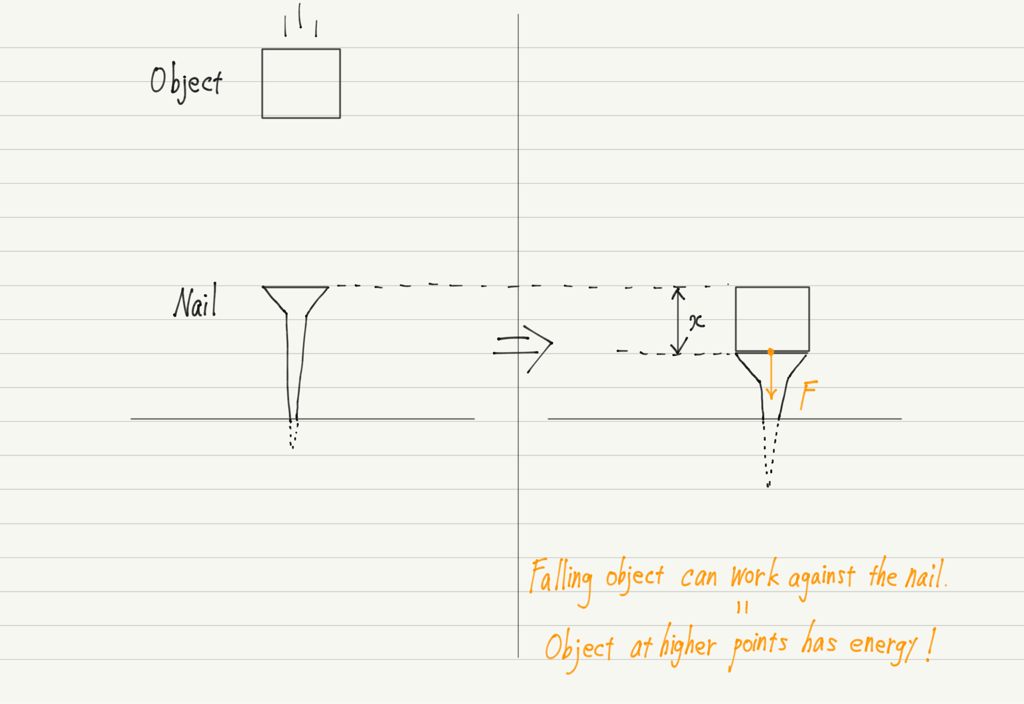Therefore, I would like to focus on the calculation of potential energy.

## Formula for gravitational potential energy

The higher the position of an object, the more potential energy it has.
How much difference is there between the potential energy of a 10 kg object at a height of 5 m from the ground and at a height of 10 m?
While introducing the formula, we will actually try to find it.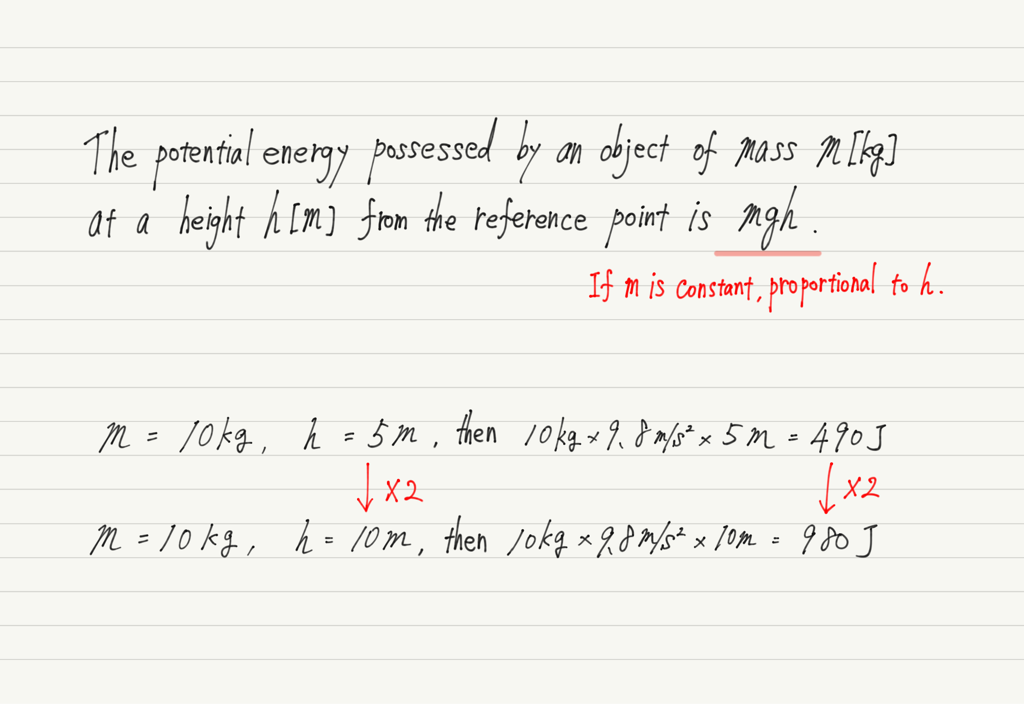Thus, when the height doubles, the potential energy also doubles, indicating a simple proportional relationship between potential energy and height.

By the way, do you remember the mgh formula from somewhere?
If you don’t remember it, please take a look at the following article.

https://www.yukimura-physics.com/entry/dyn-f25

The mgh was the work done by gravitational force.
Gravitational force does mgh work on an object until it falls and reaches the ground.

This work done by gravitational force is converted into another work (in the above example, the work of driving a nail into the ground), so we can say that the true nature of potential energy is the work done by gravitational force.

## Reference point　for potential energy

The school I attended had a laboratory on the fourth floor.
Suppose we are going to conduct a potential energy experiment in this laboratory.

Let us calculate the potential energy mgh.
m can be found quickly using a scale. Since g is the gravitational acceleration, we substitute 9.8m/s2.

Finally, the height h. Where is the height measured from?

When we say “an object is at a high place,” I think everyone unconsciously imagines its height from the ground.
Of course, that’s not wrong, but in this case, measuring the height from the ground would be a challenge!
Because the laboratory is on the fourth floor!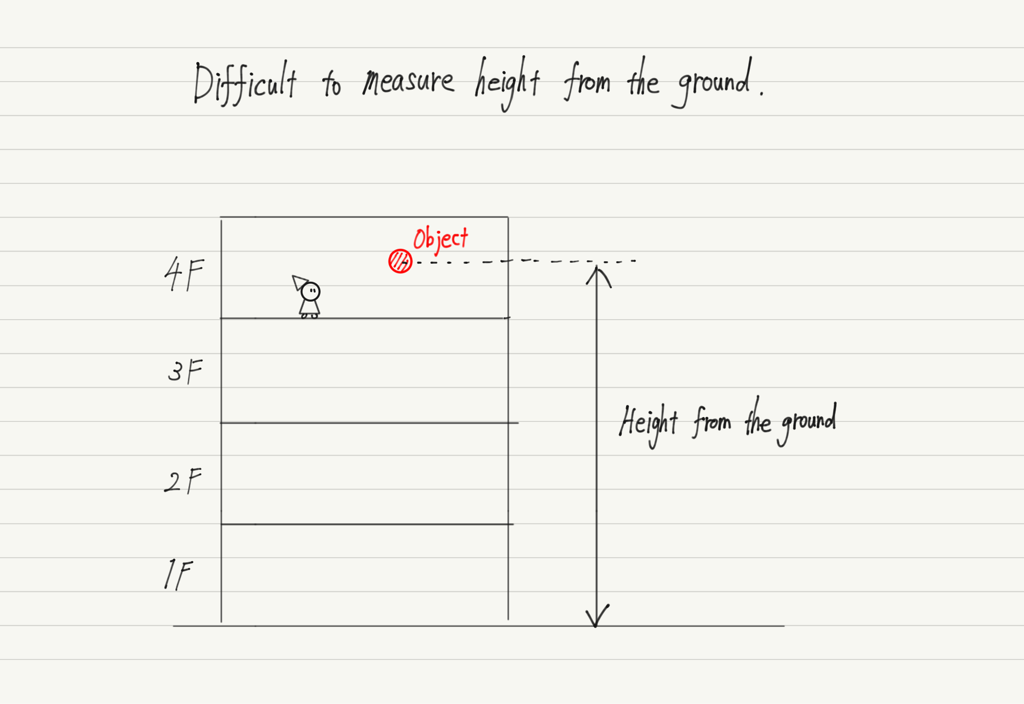But if you think about it, when you drop an object in the laboratory, it does not fall to the ground. It ends up on the floor.
The fact that the object does not fall down from the floor means that the potential energy should be zero when the object is on the floor.

In other words, the fourth floor is used as the reference point  for potential energy.

When calculating potential energy in this way, the reference point for measuring height can be determined freely, depending on the situation!

Also, since the height from the reference point depends on where the reference point is set, it is possible that the potential energy of the same object may have different values for person A and person B.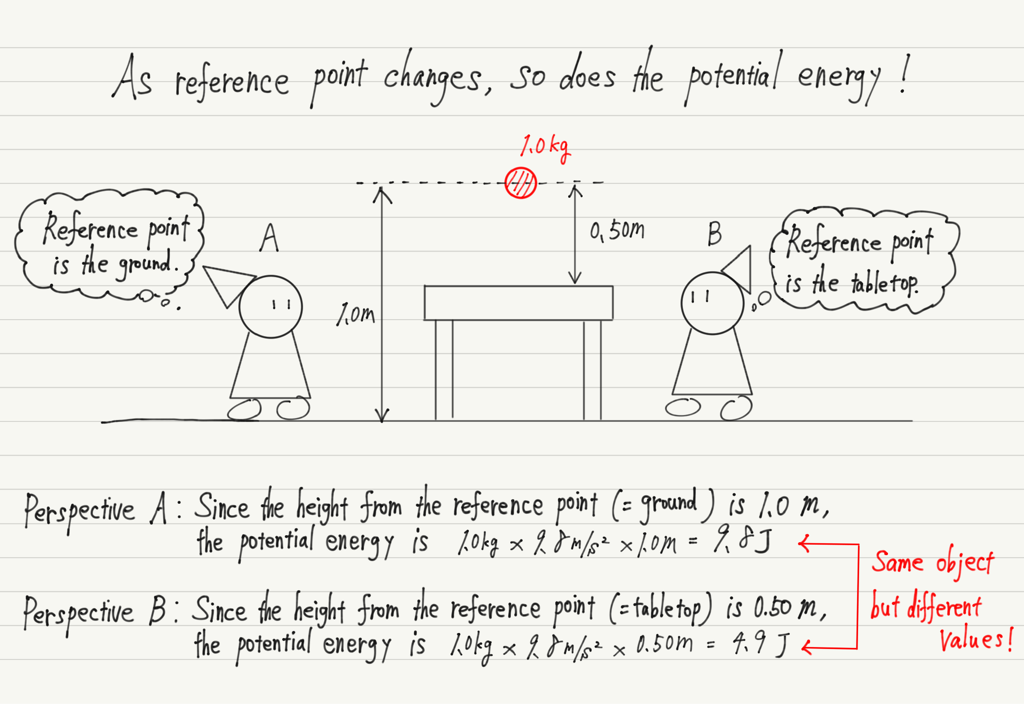Even though the potential energy is different, the “result of the calculation using the potential energy (e.g., the velocity of the fall)” remains the same, so you can rest assured.

## When an object is below the reference　point

It is very convenient to be free to determine the reference point, but at the same time it creates certain adverse effects.
That is “the possibility of an object being below the reference point”.

If it is based on the ground, the possibility is zero, but if it is based on the fourth floor, as in the previous example, what about the objects on the third floor?

This problem is nicely solved by setting “lower than reference point = negative height”.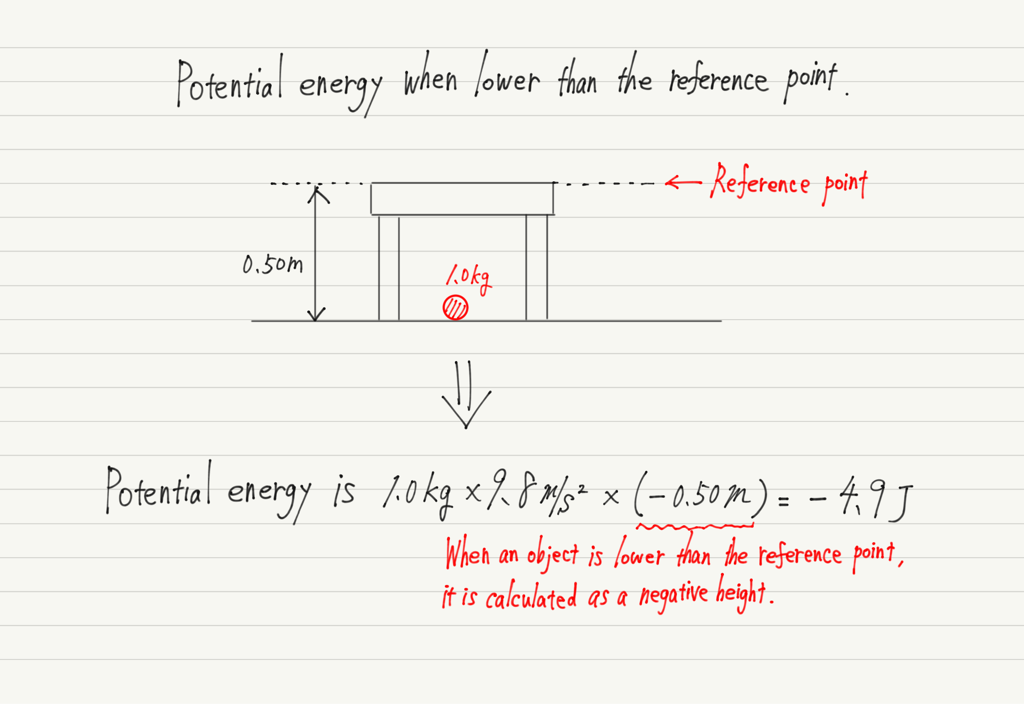Thus, objects that are lower than the reference point should be interpreted as having negative potential energy.

## Summary of this lecture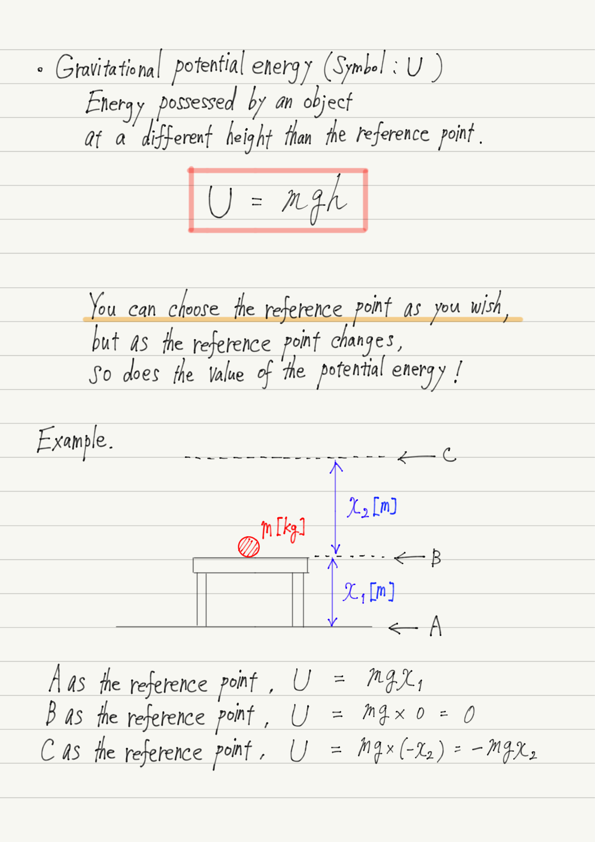## Next Time

In the next lecture, we will introduce potential energy other than gravity.

https://www.yukimura-physics.com/entry/dyn-f28

error: Content is protected !!